GeeksforGeeks App
Open AppBrowser
Continue

# Using Matplotlib with Jupyter Notebook

The Jupyter Notebook is an open-source web application that allows you to create and share documents that contain live code, equations, visualizations and narrative text. Uses include data cleaning and transformation, numerical simulation, statistical modeling, data visualization, machine learning, and much more. Note: For more information, refer to How To Use Jupyter Notebook – An Ultimate Guide Matplotlib is one of the most popular Python packages used for data visualization. It is a cross-platform library for making 2D plots from data in arrays.To get started you just need to make the necessary imports, prepare some data, and you can start plotting with the help of the plot() function.When you’re done, remember to show your plot using the show() function. Matplotlib is written in Python and makes use of NumPy, the numerical mathematics extension of Python.It consists of several plots like:

• Line
• Bar
• Scatter
• Histogram
• And many more

#### Installation

• Install Matplotlib with pip Matplotlib can also be installed using the Python package manager, pip. To install Matplotlib with pip, open a terminal window and type:
`pip install matplotlib`
• Install Matplotlib with the Anaconda Prompt Matplotlib can be installed using with the Anaconda Prompt. If the Anaconda Prompt is available on your machine, it can usually be seen in the Windows Start Menu. To install Matplotlib, open the Anaconda Prompt and type:
`conda install matplotlib`
•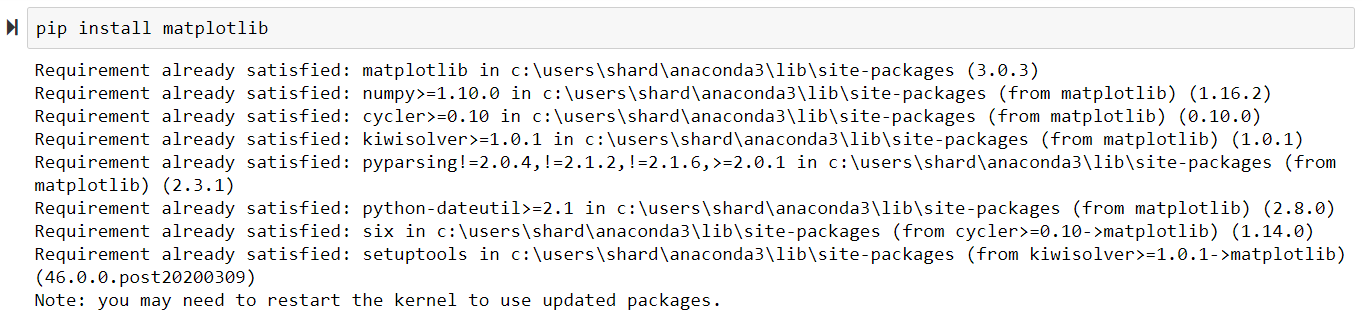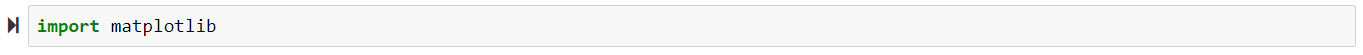## Using Matplotlib with Jupyter Notebook

After the installation is completed. Let’s start using Matplotlib with Jupyter Notebook. We will be plotting various graphs in the Jupyter Notebook using Matplotlib.

## Python3

 `# importing matplotlib module``from` `matplotlib ``import` `pyplot as plt` `# x-axis values``x ``=` `[``5``, ``2``, ``9``, ``4``, ``7``]` `# Y-axis values``y ``=` `[``10``, ``5``, ``8``, ``4``, ``2``]` `# Function to plot``plt.plot(x, y)` `# function to show the plot``plt.show()`

Output: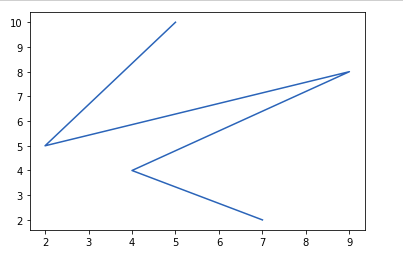Bar Plot

## Python3

 `# importing matplotlib module``from` `matplotlib ``import` `pyplot as plt` `# x-axis values``x ``=` `[``5``, ``2``, ``9``, ``4``, ``7``]` `# Y-axis values``y ``=` `[``10``, ``5``, ``8``, ``4``, ``2``]` `# Function to plot``plt.bar(x, y)` `# function to show the plot``plt.show()`

Output: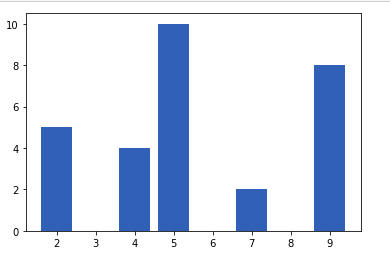Histogram

## Python3

 `# importing matplotlib module``from` `matplotlib ``import` `pyplot as plt` `# Y-axis values``y ``=` `[``10``, ``5``, ``8``, ``4``, ``2``]` `# Function to plot histogram``plt.hist(y)` `# Function to show the plot``plt.show()`

Output :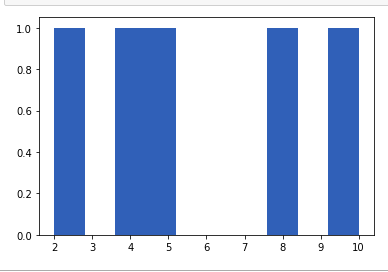## Python3

 `# importing matplotlib module``from` `matplotlib ``import` `pyplot as plt` `# x-axis values``x ``=` `[``5``, ``2``, ``9``, ``4``, ``7``]` `# Y-axis values``y ``=` `[``10``, ``5``, ``8``, ``4``, ``2``]` `# Function to plot scatter``plt.scatter(x, y)` `# function to show the plot``plt.show()`

Output :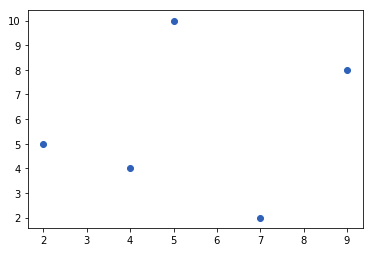#### Adding title and Labeling the Axes in the graph

We can add title to the graph by using the following command

`matplotlib.pyplot.title("My title")`

We can label the x-axis and y-axis by using the following functions

```matplotlib.pyplot.xlabel("Time (Hr)")

matplotlib.pyplot.ylabel("Position (Km)")```

Example :

## Python3

 `# importing matplotlib module``from` `matplotlib ``import` `pyplot as plt` `# x-axis values``x ``=` `[``5``, ``2``, ``9``, ``4``, ``7``]` `# Y-axis values``y ``=` `[``10``, ``5``, ``8``, ``4``, ``2``]` `# Function to plot``plt.scatter(x, y)` `# Adding Title``plt.title("GeeksForGeeks")` `# Labeling the axes``plt.xlabel("Time (hr)")``plt.ylabel("Position (Km)")` `# function to show the plot``plt.show()`

Output: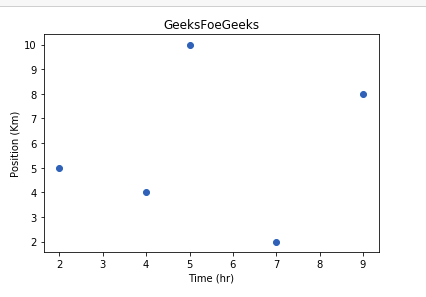We can also write a program in the same cell for printing Multiple Graphs together. We can print these graphs vertically one below another by repeating the show() function in the program or we can use a function called subplot() in order to print them horizontally as well.

## Python3

 `from` `matplotlib ``import` `pyplot as plt`  `x ``=` `[``1``, ``2``, ``3``, ``4``, ``5``]``y ``=` `[``1``, ``4``, ``9``, ``16``, ``25``]``plt.scatter(x, y)` `# function to show the plot``plt.show()` `plt.plot(x, y)` `# function to show the plot``plt.show()`

Output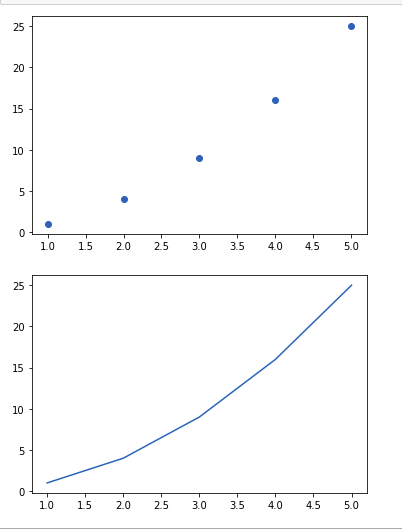My Personal Notes arrow_drop_up